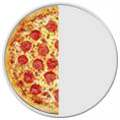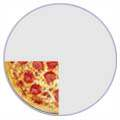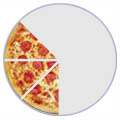• If you are citizen of an European Union member nation, you may not use this service unless you are at least 16 years old.

• Want to get organized in 2022? Let Dokkio put your cloud files (Drive, Dropbox, and Slack and Gmail attachments) and documents (Google Docs, Sheets, and Notion) in order. Try Dokkio (from the makers of PBworks) for free. Available on the web, Mac, and Windows.

View

Mathtastic!

last edited by 9 years, 11 months ago

Mathtastic!

What is a Fraction?

Fractions

A fraction is a part of a whole

Slice a pizza, and you will have fractions:1/2 1/4 3/8 (One-Half) (One-Quarter) (Three-Eighths) The top number tells how many slices you have The bottom number tells how many slices the pizza was cut into.

Numerator / Denominator

We call the top number the Numerator, it is the number of parts you have.
We call the bottom number the Denominator, it is the number of parts the whole is divided into.

You just have to remember those names! (If you forget just think "Down"-ominator)

Numerator

Denominatator

Here is an online lesson with activity!

Part 1:

Equivalent Fractions:

Definition:  Fractions that name the same amount. example 1/2 and 2/4

*Remember equivalent means equal but different!Whatever you do the top (multiply or divide only) you must do to the bottom

Review Activities:

Fraction Frenzy (must have adobe shockwave to run)

Matching Equivalent Fractions (uses adobe Flash)

Melvin's Make a Match (a cyberchase game)

Fresh Baked Fractions (Funbrain)

Equivalent Fraction Shoot (several skill levels)

Fractions: "Simplest Form"

Definition: a fraction is in "Simplest Form" when the numerator and denominator have only 1 common factor.

* remember that a factor is a Number that divides a given number exactly

example the factors of 12 are 1;2;3;4;6;12

Review Activities:

Simplify the Fraction (Java game. takes awhile to load)

aaaknow game (includes review lesson and variety of level for game)

Math Playground (includes review lesson)

Soccer Math (math-play.com)

Reduce Fractions Shoot (Reducing and simpliest form)

Find Grampy

Part 2:

Mixed Numbers:

Definition: a mixed number includes a Whole number and a Fraction  ex. 3 1/3

Review activities:

The Birds (Java game. Does not require "simplest form")

Math Playground (includes review lesson)

Clara's Ice Cream Shop (timed)

Matching Mixed Numbers

Compare and Order Fractions & Mixed Numbers:

Ways to Compare and Order:1) Use fraction bars

2) Rename fractions with unlike denominators with like denominators

3) Convert into a decimal, then compare (coming soon!)

Review Activities:

Balloon Pop Math

Skillwise  (includes lesson, games, visual aides, and quizzes)

Number Line Activity

Fun Video (Careful the song is catchy!)Part 3:

Relate Fractions to Decimals:To change a fraction to a decimal simply: 1) Write down the whole followed by a decimal point

2) Divide the numerator by the denominator (using a calculator)

3) Write everything after your decimal point on the calculator

after the decimal on your paper

Review Activities:

aaamath (includes review lesson and variety of level for game)

Tank game (toon university conversion game)

Death to Decimals! (fun but challenging similar to Space Invaders)

Fraction Four (Connect Four make sure you change the time and pick fraction to decimals)

Three Games (Concentration, flashcards, or Matching uses java)

Part 4:To add or subtract like fractions: 1) make the denominator the same

3) Simplify

Review Activities:

Fruit Shoot (Levels 1 and 2 have like denominators)

Island Math (Click on "too hard" to receive problems that have like denominators. uses java)

Action Fraction (Car race)To add or subtract unlike fractions: 1) Make the denominator the same. To do this you must:

a) rewrite the problem

b) find the LCM of the denominators (LCM review lesson)

c) use the LCM as the denominator for each fraction in

2) Write equivalent fractions to determine the numerators

4) Simplify

Decimals

Compare and order Decimals:

*Remember to make equivalent decimals before trying to order them!  If you need a review lesson click here.

For a worksheet to show work click .Back to Frontpage